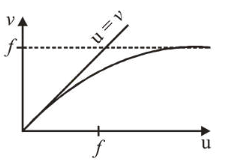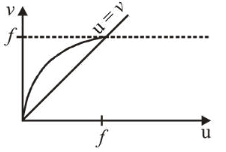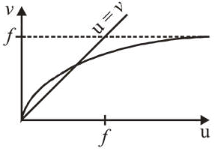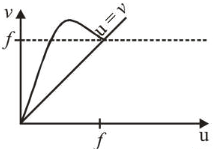# For a concave lens of focal length f,

Question:

For a concave lens of focal length $f$, the relation between object and image distances $u$ and $v$, respectively, from its pole can best be represented by ( $u=v$ is the reference line):

1.2.3.4.Correct Option: 4

Solution:

(4) From lens formula,

$\frac{1}{v}-\frac{1}{u}=\frac{1}{f} \Rightarrow v=\frac{u f}{u+f}$

Case-I : If $v=u \Rightarrow f+u=f \Rightarrow u=0$

Case-II : If $u=\infty$ then $v=f$.

Hence, correct $u$ versus $v$ graph, that satisfies this condition is (a).